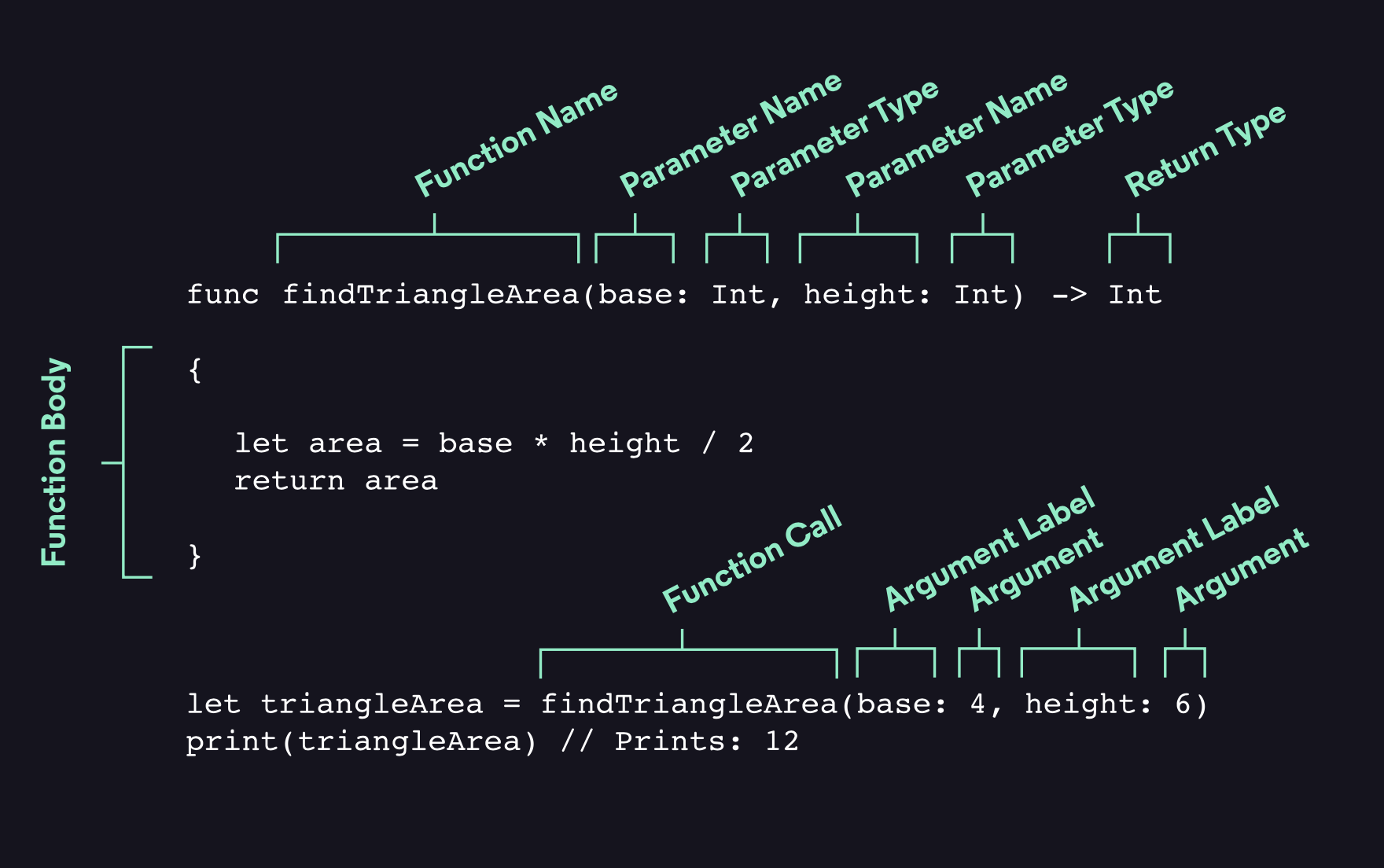# Functions

Functions are blocks of code organized together to perform a specific task. A function is given a name that identifies what it does, and this name is used to “call” the function to perform its task when needed.

## How to Define and Call a Function

A function has three important elements that define it. First is the function name, which is used to call the function. Second are the parameters a function takes, parameters refer to the input the function takes when it is called. Lastly is the return type of the function, which refers to the output data type of the function. When defining the name of the function, the name should describe its functionality.

The figure below describes the syntax of functions in Swift:```func salute(name: String) -> String {    let salute = "Hello, " + name + "!"    return salute}
```

For example, the function `salute` takes a `String` parameter called `name`, and returns a `String` equal to “Hello” plus the `name` value. The `salute()` function is called by passing it a `String` value after the `name:` argument label as shown below:

```print(salute(name: "Anna"))// Prints "Hello, Anna!"
```

## Multiple Parameters

Functions can take more than one input parameter, the parameters must be separated by commas and assigned during the function call.

For example, this function takes two numbers as an input, and returns the sum of the two numbers:

```func sum(x: Int, y: Int) -> Int {  return x + y}
let result = sum(x: 1, y: 2)print(result) // Prints:  3
```

## Returning Multiple Values

A tuple can be used as the return type for a function to allow for multiple return values. Each value within the parentheses must be labeled and assigned a type in the function definition.

For example, this function takes array of `Int` as an input, and returns the smallest and largest number in the array.

```func minMax(array: [Int]) -> (MinNumber: Int, MaxNumber: Int) {    var MinNumber = array    var MaxNumber = array    if array.isEmpty {    return nil    }else{    for value in array {        if value < MinNumber {            MinNumber = value        } else if value > MaxNumber {            MaxNumber = value        }    }    }    return (MinNumber, MaxNumber)}
```

Because the tuple’s member values are named as part of the function’s return type, they can be accessed with dot syntax to retrieve the minimum and maximum found values, as done below:

```let rangeVals = minMax(array: [12, 11, -2, 327, 230, 95])print("Minimum number is \(rangeVals.MinNumber) and maximum number is \(rangeVals.MaxNumber)")// Prints "Minimum number is -2 and maximum number is 327"
```

## How to Omit Argument Labels

To avoid having an argument labeled, use the `_` instead of an explicit argument label for that parameter.

```func findDifferenceBetween(_ a: Int, _ b: Int) -> Int {  return a - b}
print(findDifferenceBetween(6,4)) // Prints: 2
```

Note: If a parameter has an argument label, the argument must be labeled when calling the function.

## An Implicit Return

If the whole function body consists of a single expression, the function implicitly returns the value of that expression. For example:

```func salute(name: String) -> String {  "Hello, " + name + "!"}
```

The entire definition of the `salute()` function is the message that it returns, which means it can use this shorter form. Any function that contains one return line can omit the `return` keyword.

## Default Parameters

A default parameter has a value assigned to it in the function’s definition. For example:

```func timeToFinishBook(numWords: Double, wordsPerMin: Double = 200) -> Double {  let totalMinutes = numWords / wordsPerMin  return totalMinutes / 60}
print("\(timeToFinishBook(numWords: 93000)) hours")// Prints: 7.75 hours
```

When a function with a default parameter is called, an argument for that parameter is not required. If the argument is included, that value will overwrite the default value and be used in the function body.

## How to Use Variadic Parameters

Variadic parameters are flexible arguments that accepts zero or more values of a specified type. They are declared by inserting three period characters (`...`) after the parameter’s type name. The example below calculates the sum of all the elements in a list of numbers of any length:

```func totalSumof(_ numbers: Int...) -> Int {    var total: Int = 0    for number in numbers {        total += number    }    return total}totalSumof(1, 2, 3, 4, 5)// returns 15, which is the sum of the numbers
```

The values passed to a variadic parameter are made available within the function’s body as an array. For example, a variadic parameter with a name of `numbers` and a type of `Int...` is made available within the function’s body as a constant array called `numbers` of type `Int`.

## Functions With In-Out Parameters

To be able to change the value passed for a certain argument, an `inout` argument should be used. An in-out parameter is denoted with the `inout` keyword in the function definition, and when the function is called, its variable argument must be prepended with an `&`.

Note: Parameters declared as `inout` can’t have default values, and variadic parameters can’t be marked as `inout`.

```func decrement(_ a: inout Int) {    a -= 1}
```

This function decrements a passed value by 1.

```var number = 3decrement(&number)print(number)// Prints 2
```

The example above shows that the original value of `number` is modified by the `decrement()` function, even though the variable was originally defined outside of the function.

Note: In-out parameters aren’t the same as returning a value from a function. The `decrement()` example above doesn’t define a `return` type or return a value, but it still modifies the value of `number`. In-out parameters are an alternative way for a function to have an effect outside of the scope of the function body.